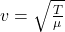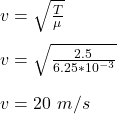## The mass of a string is 20 g and it has a length of 3.2 m. Assuming that the tension in the string is 2.5 N, what will be the wavelength of

Question

The mass of a string is 20 g and it has a length of 3.2 m. Assuming that the tension in the string is 2.5 N, what will be the wavelength of a travelling wave that is created by a sinusoidal excitation of this string with a frequency of 20 Hz. Provide the wavelength in units of m. Please note: You do not include the units in your answer. Just write in the number.

in progress 0
2 months 2021-07-22T14:14:37+00:00 1 Answers 2 views 0

The wavelength of the wave is 1 m

Explanation:

Given;

mass of the string, m = 20 g = 0.02 kg

length of the string, L = 3.2 m

tension on the string, T = 2.5 N

the frequency of the wave, f = 20 Hz

The velocity of the wave is given by;where;

μ is mass per unit length = 0.02 kg / 3.2 m

μ = 6.25 x 10⁻³ kg/mThe wavelength of the wave is given by;

λ = v / f

λ = (20 m/s )/ (20 Hz)

λ = 1 m

Therefore, the wavelength of the wave is 1 m